Register
 Email: Passwd 验 证:换一张 Have you forgotten your password? Stay signed in Log In
 ALL Title Author Keyword Sponsors Type Publication date Submitted Time Subject Conference Name Source Categories KOS Subject Advisor ORCID Advanced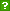ISCAS OpenIR  > 中科院软件所  > 中科院软件所
 Title: 网络优化问题的近似算法 Author: 张鹏 Issued Date: 2007-06-08 Degree Grantor: 中国科学院软件研究所 Place of Degree Grantor: 软件研究所 Degree Level: 博士 Keyword: 网络优化 ; 网络设计 ; 割集 ; 近似算法 ; 组合优化 Alternative Title: Approximation Algorithms for Network Optimization Problems Abstract: 新世纪信息技术和软件产业的一个显著的特征是计算机在网络环境中工作,依靠底层的通信链路交换信息. 这就自然产生了越来越多的网络优化问题. 这些问题通常是大规模的,需要快速求解. 许多的网络优化问题被证明是NP难的. 然而,我们必须在实践中处理这些问题. 近似是处理NP难优化问题的一种成功的方法,近似算法致力于在多项式时间内给出问题的一个尽可能最优的解.对近似算法的研究不但是越过问题的NP困难性的一种方式, 也是求解NP难优化问题的本质的方法. 我们使用近似方法研究网络优化问题,对这些问题给出近似算法和证明问题的可近似性下界. 有两大类典型的网络优化问题.一类是网络设计问题(Network Design),这类问题关注的目标是将若干源－目标对(或终端集)连通起来. 另一类是割集问题(Cut),这类问题关注的目标是将若干源－目标对(或终端集)断开. 从问题目标上看它们是相互对偶的.网络设计问题和割集问题在计算机网络、电信网络、运输流网络和集成电路设计等方面有着广泛的应用.具体而言, 我们主要研究了如下四个方面的问题, 它们是两类网络优化问题中的典型代表. 一、k-Facility Location问题(选址问题).给定若干设施位置点和需求点以及它们之间的距离, k-Facility Location问题询问如何以最小的费用在设施位置点打开最多不超过k个设施以满足所有需求点的需求. 该问题是运筹学中的一个经典问题. 使用局部搜索方法,对k-Facility Location问题给出了新的近似算法. 通过对解的结构的深入分析,证明算法的近似比为2 + \sqrt{3} + \epsilon. 该结果是关于k-Facility Location问题目前已知最好的近似比. 二、Min-Sum和Min-Max不相交路径问题(选路问题). Min-Sum不相交路径问题询问问题的一个解, 连通所有的需求(源－目标对),使所选取路径的总长度最短. Min-Max不相交路径问题询问连通所有需求的解, 使所选取路径中最长路径的长度最短. 在复杂性方面, 证明了一般图上的Min-Sum不相交路径问题是FP^NP完全的. 在不可近似性方面, 证明了只要P \neq NP, 对任意小的常数\epsilon > 0, 即使在平面图上Min-Sum不相交路径问题和Min-Max不相交路径问题不能够被近似到\Omega(m^{1-\epsilon})以内, 其中m是图上边的数目.以上复杂性结果和不可近似性结果推广了前人的结果, 并且这些结果是更强的,因为它们是在即使已知输入实例是可行的(即,已知输入实例存在连通全部需求的解)条件下被证明的. 基于线性规划技术,对Min-Max边不相交路径问题和Min-Sum边不相交路径问题首次给出了Bicriteria近似算法. 三、k-Steiner Forest问题(网络连通性问题). 给定图上l个需求(源－目标对),k-Steiner Forest问题询问费用最小的解, 连通其中至少k个需求.该问题是Hajiaghayi和Jain在SODA'06上提出的一个有趣的未解决问题.证明了贪心算法的一个复合引理, 并由该引理推导出了k-Steiner Forest问题的一个贪心算法,其近似比为O(\min { n^{2/3}, \sqrt l} \log k), 改进了关于此问题文献已知最好近似比.并且, 贪心算法的复合引理具有其一般性, 可用于近似一类部分覆盖(Partial Cover)类型的优化问题. 四、树上推广的Multicut问题(割集问题). 提出并研究了树上推广的Multicut问题.给定树上l个终端集, 树上推广的Multicut问题询问费用最小的一组边,使得在树上删除这组边能够断开所有的终端集.对该问题的Prize-collecting版本给出了近似比为3的原始－对偶近似算法和近似比为2.55的随机化近似算法, 对该问题的k-版本给出了近似比为min{ 2(l-k+1), k}的近似算法.找到了k版本的树上推广的Multicut问题和k-稠密子图问题之间的一个有趣的联系,从而证明将k-版本的树上推广的Multicut问题近似到O(n^{1/6-\epsilon})以内是困难的,其中\epsilon > 0是某个小的常数. 最后, 我们指出改进k-Facility Location问题的近似比、设计树上k-Steiner Forest问题的近似算法以及改进树上推广的k-Multicut问题的近似比是与本文工作密切相关的几个Open问题. English Abstract: A remarkable feature of information technology and software industry in the new century is that computers work in a network environment, exchanging information through underlying telecommunications. This gives rise to more and more large-scaled network algorithmic problems. Many nontrivial network optimization problems can be proved to be NP-hard. However, we must be able to tackle these problems. Approximation is one of the successful approaches to solving these problems by giving as good as possible feasible solutions in polynomial time. The study of approximation algorithms is not only a way that we go around the NP-hardness of an optimization problem, but also an essential method that we deal with the NP-hard problems. We study the network optimization problems by approximation approach, giving approximation algorithms and proving approximation hardness for these problems. There are two main categories of network optimization problems. One is about the network design problems, and the other is about the cut problems. The network design problems focus on how to connect source-sink pairs (or terminal sets). On the other hand, the cut problems focus on how to cut source-sink pairs (or terminal sets). They are reciprocally dual from the view of the goals of the problems. The network design problems and the cut problems have many applications in the fields such as computer networks, telecommunications, transportation networks and VLSI design. Specifically, we study the following four problems, which are typical in the two classes of network optimization problems. 1. $k$-Facility Location problem (Locating Problem). Given facility locations and demand points and the distances between them, the $k$-Facility Location problem asks to open at most $k$ facilities at the given locations with minimized cost to serve the demands of all the demand points. This problem is classical in operations research. We give a new approximation algorithm to the $k$-Facility Location problem based on the local search approach. By deeper analysis on the structure of the problem solutions, we prove that the performance ratio of our algorithm is $2 + \sqrt{3} + \epsilon$ for arbitrarily small constant $\epsilon > 0$, which is the best approximation result known to this problem. 2. Min-Sum and Min-Max Disjoint Paths Problems (Path-choosing Problem). Given some demands (a.k.a. source-sink pairs), the Min-Sum Disjoint Paths problem asks a solution to connect all the demands with disjoint paths, such that the total cost of the solution is minimized, while the Min-Max Disjoint Paths problem finds a solution to connect all the demands with disjoint paths, such that the length of the longest path is minimized. In the aspect of complexity, we prove that the Min-Sum Disjoint Paths problem in general graph is ${\mathbf{FP}}^{\mathbf{NP}}$-complete. In the aspect of inapproximability, we prove that even in planar graph the Min-Sum and Min-Max Disjoint Paths problems can not be approximated within ~$\Omega(m^{1-\epsilon})$ for arbitrarily small constant $\epsilon > 0$ unless ${\mathbf{P}} = {\mathbf{NP}}$, where $m$ is the number of edges in the graph. The above complexity and inapproximability results are stronger since they are proved even the instances are known to be feasible (i.e., all the demands in the instance can be connected by disjoint paths). Based on linear program technique, we give bicriteria approximation algorithms for the Min-Max and Min-Sum Edge Disjoint Paths problems at the first time. 3. $k$-Steiner Forest Problem (Network Connectivity Problem). Given $l$ demands in the graph, the $k$-Steiner Forest problem asks to connect at least $k$ demands of them with the minimized total cost. This problem is an interesting problem proposed by Hajiaghayi and Jain in SODA'06. We prove a coupling lemma about the greedy algorithm, and thus deduce a greedy approximation algorithm for the $k$-Steiner Forest problem with performance ratio $O(\min \{ n^{2/3}, \sqrt l\} \log k)$, which improves the best ratio known to this problem in the literature. Moreover, the coupling lemma about greedy algorithm has its generality and may find its more applications in approximating some partial cover-like optimization problems. 4. Generalized Multicut in Trees (Cut Problem). Given $l$ terminal sets in the tree, the Generalized Multicut in Trees problem finds a set of edges with minimized total cost, such that removing these edges from the tree cut every terminal set. We give a primal-dual approximation algorithm with factor of 3 and a randomized approximation algorithm with factor of 2.55 for the prize-collecting version of the problem. For the $k$-version of the problem, we give a $\min \{ 2(l-k+1), k \}$-approximation algorithm. In addition, we find an interesting relation between the $k$ version of the problem and the Densest $k$-Subgraph problem, and thus prove that it is hard to approximate the $k$-Generalized Multicut in Trees problem within $O(n^{1/6-\epsilon})$ for some small constant $\epsilon > 0$. At last, we point out that improving performance ratio for the $k$-Facility Location problem, designing approximation algorithm for the $k$-Steiner Forest in Trees problem and improving performance ratio for the generalized $k$-Multicut in Trees problem are important open problems closely related to this thesis. Language: 中文 Content Type: 学位论文 URI: http://ir.iscas.ac.cn/handle/311060/5710 Appears in Collections: 中科院软件所

 Files in This Item:
File Name/ File Size Content Type Version Access License
10001_200418015029023张鹏_paper.pdf（1336KB）----限制开放-- 联系获取全文

 Recommended Citation: 张鹏. 网络优化问题的近似算法[D]. 软件研究所. 中国科学院软件研究所. 2007-06-08.
 Service Recommend this item Sava as my favorate item Show this item's statistics Export Endnote File Google Scholar Similar articles in Google Scholar [张鹏]'s Articles CSDL cross search Similar articles in CSDL Cross Search [张鹏]‘s Articles Related Copyright Policies Null Social Bookmarking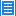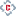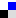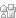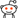您对该条目有什么异议，请填写以下表单，管理员会尽快联系您。 内 容： Email： * 单位： 验证码：刷新
 您在IR的使用过程中有什么好的想法或者建议可以反馈给我们。 标 题： * 内 容： Email： * 验证码：刷新Copyright © 2007-2021  中国科学院软件研究所 - Feedback Powered by CSpace